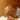# Modules - Python practice book

1/4/2018, 8:16:00 PM

1. Write a program to list all files in the given directory.
``````import os
def listdir(dir, handler):
with os.scandir(dir) as files:
for file in sorted(
files,
key=lambda file:file.stat().st_size,
reverse=True
):
handler(file)
listdir(os.getcwd(), lambda file:print(file.name))``````
1. Write a program `extcount.py` to count number of files for each extension in the given directory. The program should take a directory name as argument and print count and extension for each available file extension.
``````cache = dict()
def statisticext(file, mem = cache):
if file.is_file():
name, ext = os.path.splitext(file.name)
if ext in mem:
mem[ext] += mem[ext]
else:
mem[ext] = 1

listdir(os.getcwd(), statisticext)

for ext, count in cache.items():
print('{count:<4} {ext:<10}'.format(ext=ext, count=count))``````
1. Write a program to list all the files in the given directory along with their length and last modification time. The output should contain one line for each file containing filename, length and modification date separated by tabs.
``````import math

def convert(bytes, lst = ['Bytes', 'KB', 'MB', 'GB', 'TB', 'PB']):
i = 0 if bytes < 1024 else int(math.floor(math.log(bytes, 1024)))
l = len(lst)
if i >= l:
i = l - 1
return '{value:.2f} {strlst}'.format(
value = bytes/math.pow(1024, i),
strlst = lst[i]
)

def formatfilelist(file):
stat = file.stat()
print('{name:>50}\t{length:>20}\t{modifytime}'.format(
name = file.name,
length = convert(stat.st_size),
modifytime = time.strftime(
'%Y/%m/%d %H:%M:%S',
time.localtime(stat.st_mtime)
)
))

listdir(os.getcwd(), formatfilelist)``````
1. Write a program to print directory tree. The program should take path of a directory as argument and print all the files in it recursively as a tree.
``````import time
sep = '/'
def tree(dir, layer = 0, base = ''):
if base == '':
base = os.path.basename(dir)
if layer == 0:
print(base + sep)
else:
print('|  ' * (layer - 1) + '|--' + base + sep)
with os.scandir(dir) as files:
for file in sorted(files, key=lambda file:file.is_dir(), reverse=True):
if file.is_dir():
tree(file.path, layer + 1, base = os.path.relpath(file.path, dir))
else:
print('|  ' * layer + '|--' + file.name)

tree(os.getcwd())``````
1. Write a program wget.py to download a given URL. The program should accept a URL as argument, download it and save it with the basename of the URL. If the URL ends with a /, consider the basename as index.html.
``````import sys
from urllib.request import urlopen

# 依赖第三方模块
import chardet

def wget(url):
# 简单处理
filename = 'index.html' if url.endswith('/') else url[url.rfind('/') + 1:]
with urlopen(url) as res:
charset = chardet.detect(data)
f.write(data.decode(charset['encoding']))

if (__name__ == '__main__'):
print('wget:', sys.argv)
wget(sys.argv)``````
1. Write a program `antihtml.py` that takes a URL as argument, downloads the html from web and print it after stripping html tags.

2. Write a function make_slug that takes a name converts it into a slug. A slug is a string where spaces and special characters are replaced by a hyphen, typically used to create blog post URL from post title. It should also make sure there are no more than one hyphen in any place and there are no hyphens at the biginning and end of the slug.

``````import re

plist = [
'hello',
'hello world',
'hello   world',
'   --- hello-  world---'
]

def make_slug(string):
return re.sub(r'[-\s]+', '-', string.strip('- '))

print(
[make_slug(item) for item in plist]
)
``````
1. Write a program `links.py` that takes URL of a webpage as argument and prints all the URLs linked from that webpage.

2. Write a regular expression to validate a phone number.

(略)

1. Write a program `myip.py` to print the external IP address of the machine. Use the response from `http://httpbin.org/get` and read the IP address from there. The program should print only the IP address and nothing else.
``````import json
from urllib.request import urlopen

u = 'http://httpbin.org/get'
with urlopen(u) as res:
print(data.get('origin'))``````
1. Write a python program `zip.py` to create a zip file. The program should take name of zip file as first argument and files to add as rest of the arguments.

2. Write a program `mydoc.py` to implement the functionality of pydoc. The program should take the module name as argument and print documentation for the module and each of the functions defined in that module.

3. Write a program `csv2xls.py` that reads a csv file and exports it as Excel file. The prigram should take two arguments. The name of the csv file to read as first argument and the name of the Excel file to write as the second argument.

4. Create a new virtualenv and install BeautifulSoup. BeautifulSoup is very good library for parsing HTML. Try using it to extract all HTML links from a webpage.

Tag:
Python TODORedky，生活在北京(北漂)，程序员，宅，喜欢动漫(海贼王)。"年轻骑士骑马出城，不曾见过绝望堡下森森骸骨，就以为自己可以快意屠龙拯救公主。"